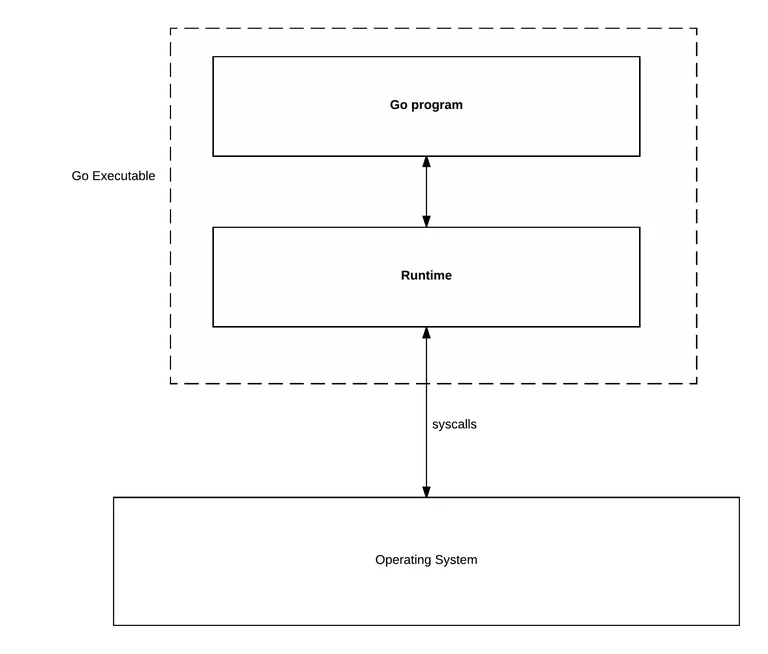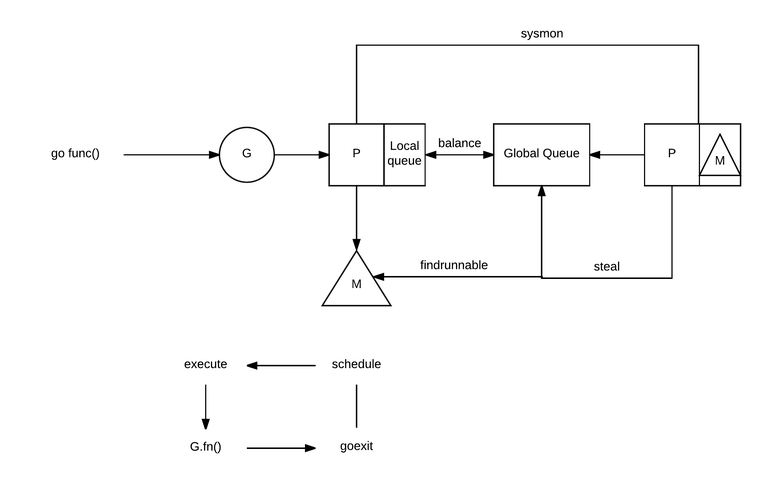# Go 调度程序：Ms，Ps&Gs## 1 基础

Go 运行时管理调度、垃圾收集和 goroutines 的运行时环境。在这里，我将只关注调度程序。## 2 Ms，Ps&Gs 之间的舞蹈

Ms、Ps 和 Gs 之间的交互有点复杂。看一下这个工作流程图：• 阻塞系统调用（例如打开一个文件），
• 网络输入，
• 通道操作，
• 同步包中的原语。

## 3 调度程序跟踪

Go 允许跟踪运行时调度程序。这是通过 GODEBUG 环境变量完成的：

$GODEBUG=scheddetail=1,schedtrace=1000 ./program 下面是它给出的输出示例： 复制代码 SCHED 0ms: gomaxprocs=8 idleprocs=7 threads=2 spinningthreads=0 idlethreads=0 runqueue=0 gcwaiting=0 nmidlelocked=0 stopwait=0 sysmonwait=0 P0: status=1 schedtick=0 syscalltick=0 m=0 runqsize=0 gfreecnt=0 P1: status=0 schedtick=0 syscalltick=0 m=-1 runqsize=0 gfreecnt=0 P2: status=0 schedtick=0 syscalltick=0 m=-1 runqsize=0 gfreecnt=0 P3: status=0 schedtick=0 syscalltick=0 m=-1 runqsize=0 gfreecnt=0 P4: status=0 schedtick=0 syscalltick=0 m=-1 runqsize=0 gfreecnt=0 P5: status=0 schedtick=0 syscalltick=0 m=-1 runqsize=0 gfreecnt=0 P6: status=0 schedtick=0 syscalltick=0 m=-1 runqsize=0 gfreecnt=0 P7: status=0 schedtick=0 syscalltick=0 m=-1 runqsize=0 gfreecnt=0 M1: p=-1 curg=-1 mallocing=0 throwing=0 preemptoff= locks=1 dying=0 helpgc=0 spinning=false blocked=false lockedg=-1 M0: p=0 curg=1 mallocing=0 throwing=0 preemptoff= locks=1 dying=0 helpgc=0 spinning=false blocked=false lockedg=1 G1: status=8() m=0 lockedm=0 注意，它使用了与 G、M 和 P 以及它们的状态相同的概念，比如 P 的队列大小。通常，你不需要那么多的细节，所以你可以使用： 复制代码 $ GODEBUG=schedtrace=1000 ./program

## 总结

https://mp.weixin.qq.com/s/wq-nirhGJ6Wm076gIN6FNw